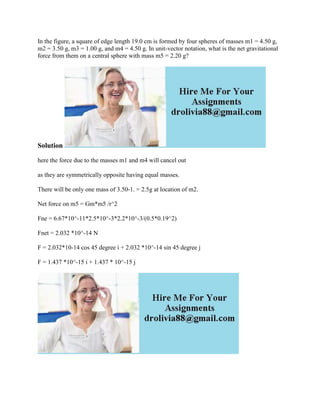Se está descargando tu SlideShare. ×

# In the figure- a square of edge length 19-0 cm is formed by four spher.docx

Anuncio
Anuncio
Anuncio
Anuncio
Anuncio
Anuncio
Anuncio
Anuncio
Anuncio
Anuncio
AnuncioCargando en…3
×

1 de 1 Anuncio

# In the figure- a square of edge length 19-0 cm is formed by four spher.docx

In the figure, a square of edge length 19.0 cm is formed by four spheres of masses m1 = 4.50 g, m2 = 3.50 g, m3 = 1.00 g, and m4 = 4.50 g. In unit-vector notation, what is the net gravitational force from them on a central sphere with mass m5 = 2.20 g?
Solution
here the force due to the masses m1 and m4 will cancel out
as they are symmetrically opposite having equal masses.
There will be only one mass of 3.50-1. = 2.5g at location of m2.
Net force on m5 = Gm*m5 /r^2
Fne = 6.67*10^-11*2.5*10^-3*2.2*10^-3/(0.5*0.19^2)
Fnet = 2.032 *10^-14 N
F = 2.032*10-14 cos 45 degree i + 2.032 *10^-14 sin 45 degree j
F = 1.437 *10^-15 i + 1.437 * 10^-15 j
.

In the figure, a square of edge length 19.0 cm is formed by four spheres of masses m1 = 4.50 g, m2 = 3.50 g, m3 = 1.00 g, and m4 = 4.50 g. In unit-vector notation, what is the net gravitational force from them on a central sphere with mass m5 = 2.20 g?
Solution
here the force due to the masses m1 and m4 will cancel out
as they are symmetrically opposite having equal masses.
There will be only one mass of 3.50-1. = 2.5g at location of m2.
Net force on m5 = Gm*m5 /r^2
Fne = 6.67*10^-11*2.5*10^-3*2.2*10^-3/(0.5*0.19^2)
Fnet = 2.032 *10^-14 N
F = 2.032*10-14 cos 45 degree i + 2.032 *10^-14 sin 45 degree j
F = 1.437 *10^-15 i + 1.437 * 10^-15 j
.

Anuncio
Anuncio

### In the figure- a square of edge length 19-0 cm is formed by four spher.docx

1. 1. In the figure, a square of edge length 19.0 cm is formed by four spheres of masses m1 = 4.50 g, m2 = 3.50 g, m3 = 1.00 g, and m4 = 4.50 g. In unit-vector notation, what is the net gravitational force from them on a central sphere with mass m5 = 2.20 g? Solution here the force due to the masses m1 and m4 will cancel out as they are symmetrically opposite having equal masses. There will be only one mass of 3.50-1. = 2.5g at location of m2. Net force on m5 = Gm*m5 /r^2 Fne = 6.67*10^-11*2.5*10^-3*2.2*10^-3/(0.5*0.19^2) Fnet = 2.032 *10^-14 N F = 2.032*10-14 cos 45 degree i + 2.032 *10^-14 sin 45 degree j F = 1.437 *10^-15 i + 1.437 * 10^-15 j